# Missing Characters Online Quiz

Following quiz provides Multiple Choice Questions (MCQs) related to Missing Characters. You will have to read all the given answers and click over the correct answer. If you are not sure about the answer then you can check the answer using Show Answer button. You can use Next Quiz button to check new set of questions in the quiz.Q 1 -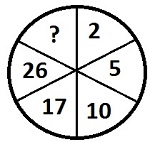Options :

A - 30

B - 36

C - 37

D - 39

### Explanation

In each step, consecutive prime numbers are added as 2 + 3 = 5, 5 + 5 = 10, 10 + 7 = 17, 17 + 9 = 26 similarly 26 + 11 = 37

Q 2 -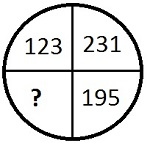Options :

A - 150

B - 156

C - 158

D - 159

### Explanation

From figure it can be seen that 231 - 36 = 195, 195 - 36 = 159 and 159 - 36 = 123. Therefore missing number is 159.

Q 3 -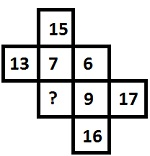Options :

A - 6

B - 9

C - 12

D - 8

### Explanation

At the end of each of rows and columns are obtain by adding the numbers in same row or column, as 6 + 7 = 13 and 6 + 9 = 15. Hence it can be conclude that 8 is the missing number.

Q 4 -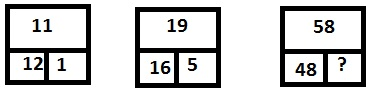Options :

A - 18

B - 19

C - 21

D - 12

### Explanation

The number at the top are obtained as, 12 + 1 - 2 = 11 and 16 + 5 - 2 = 19 similarly 48 + 12 - 2 = 58.

Q 5 -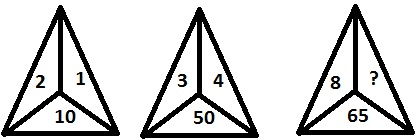Options :

A - 1

B - 3

C - 0

D - 5

### Explanation

We have 2 + 1 = 3 = 32 + 1 = 10 and 3 + 4 = 7 = 72 + 1 = 50, similarly 8 + 0 = 8 = 82 + 1 = 65. Hence missing number is 0.

Q 6 -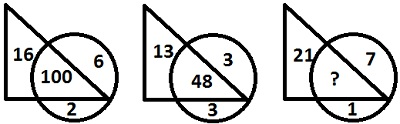Options :

A - 139

B - 145

C - 189

D - 148

### Explanation

The number at the common area of triangle and circle is obtained as, 16 × 6 + 22 = 100 13 × 3 + 32 = 48, similarly 21 × 7 + 12 = 148. Hence missing number is 148.

Q 7 -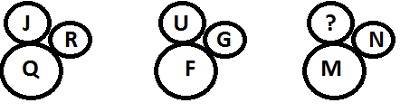Options :

A - H

B - W

C - N

D - L

### Explanation

The letters at the top has been taken opposite letter at bottom circle according to arrangement given below and letter in left to circle is following the letter at bottom.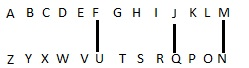Q 8 -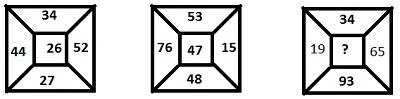Options :

A - 41

B - 23

C - 39

D - 34

### Explanation

Number at the centre = (4×4) + (5×2) = (3×4) + (2×7) = 26 according to which it is clear that 39 is the missing number as (3×4) + (3×9) = (1×9) + (6×5) = 39.

Q 9 -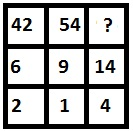Options :

A - 81

B - 76

C - 86

D - 95

### Explanation

In each column, digits of number at the top are added to get number below it and then are subtracted to get number at bottom, such as (4 + 2) = 6 and (4 - 2) = 2. In this way from given option it is clear that 95 is the missing number.

Q 10 -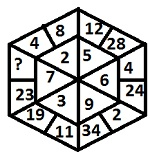Options :

A - 43

B - 44

C - 47

D - 45

In the figure all space consist of three numbers related to each other as, $\frac{8−4}{4} +1 = 2, \frac{28−12}{4} +1 = 5$, similarly 47 most be missing number which is satisfying the sequence as $\frac{47−23}{4} +1 = 7$.